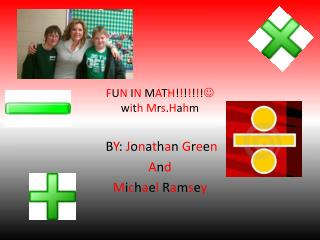DownloadDownload PresentationF U N I N M A T H ! ! ! ! ! ! !  w i t h M r s . H a h m

# F U N I N M A T H ! ! ! ! ! ! !  w i t h M r s . H a h m

Télécharger la présentation## F U N I N M A T H ! ! ! ! ! ! !  w i t h M r s . H a h m

- - - - - - - - - - - - - - - - - - - - - - - - - - - E N D - - - - - - - - - - - - - - - - - - - - - - - - - - -
##### Presentation Transcript

1. FUN IN MATH!!!!!!!withMrs.Hahm BY: JonathanGreen And Michael Ramsey

2. Circles number 1 and 2 A=3xr2 A=12in2 R=2in D=4in A=36.75in2 R=3.5in D=7in

3. Triangles numbers 1 and 2 A=B X H divided by 2 B=3in H=3in A=4.5in2 B=7in H=7in A=24.5in2

4. Rectangle 1 and 2 • A=l x w L=27in W=13in A=351 in2 L=3in W=4in A=12in2

5. Trapezoid 1 A=base1 + base 2 x height divided by 2 Base 1= 50 in Base 2=49in Height = 40in A=1,980in2

6. GO HOGS!!!!!!! • WOO PIG SOOIE